International Fixed Income Topic IVB International Fixed Income

• Slides: 23International Fixed Income Topic IVB: International Fixed Income Pricing Investment StrategiesOutline • Strategies – Relation between international fixed income bonds – Examples of active strategies • An example: mean-variance analysis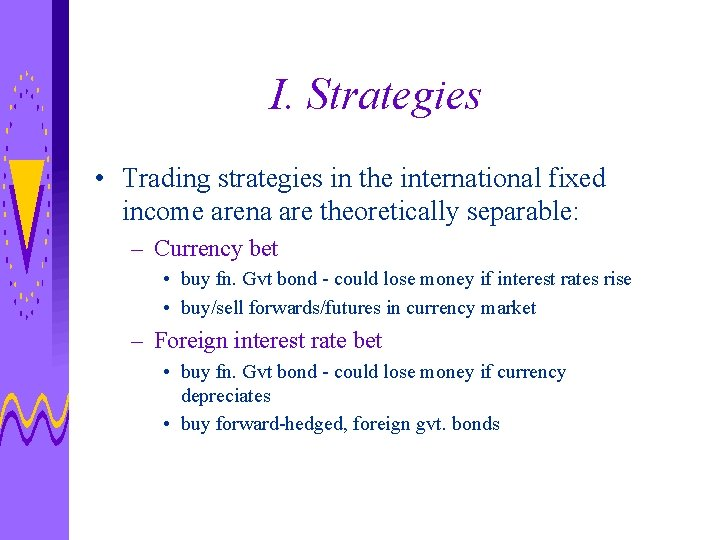I. Strategies • Trading strategies in the international fixed income arena are theoretically separable: – Currency bet • buy fn. Gvt bond - could lose money if interest rates rise • buy/sell forwards/futures in currency market – Foreign interest rate bet • buy fn. Gvt bond - could lose money if currency depreciates • buy forward-hedged, foreign gvt. bonds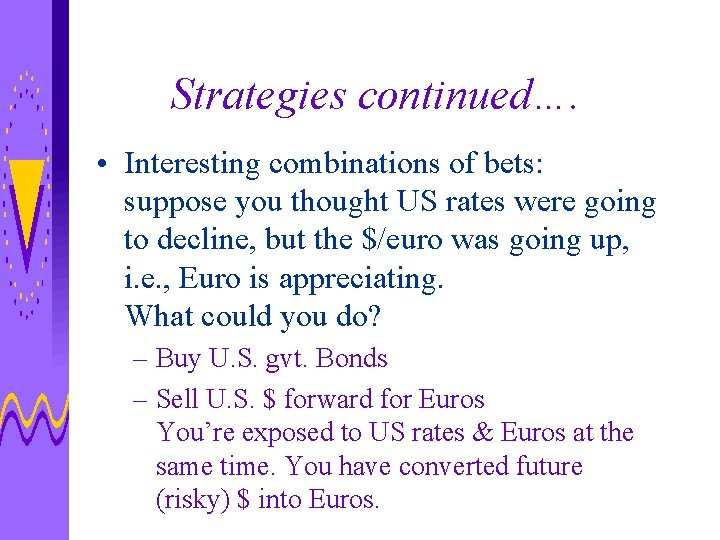Strategies continued…. • Interesting combinations of bets: suppose you thought US rates were going to decline, but the \$/euro was going up, i. e. , Euro is appreciating. What could you do? – Buy U. S. gvt. Bonds – Sell U. S. \$ forward for Euros You’re exposed to US rates & Euros at the same time. You have converted future (risky) \$ into Euros.IA: Pricing International Fixed Income Bonds • How do you price future cash flows? • What does interest rate parity tell us about relative discount factors across countries? • General pricing formula • Example from class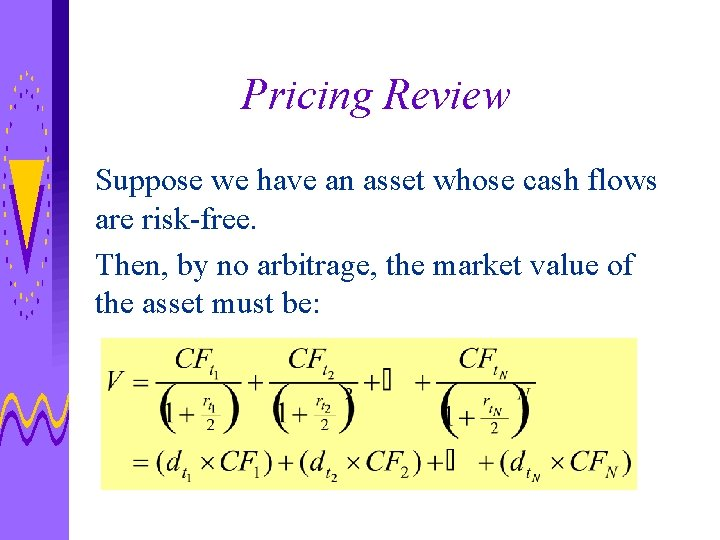Pricing Review Suppose we have an asset whose cash flows are risk-free. Then, by no arbitrage, the market value of the asset must be: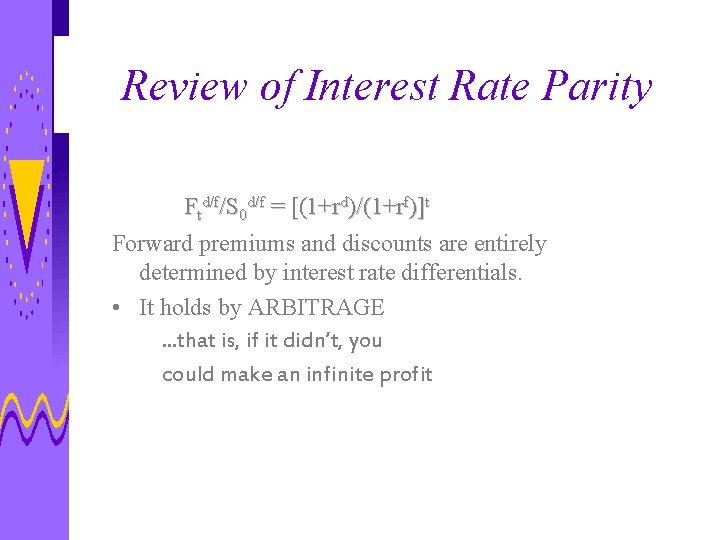Review of Interest Rate Parity Ftd/f/S 0 d/f = [(1+rd)/(1+rf)]t Forward premiums and discounts are entirely determined by interest rate differentials. • It holds by ARBITRAGE. . . that is, if it didn’t, you could make an infinite profit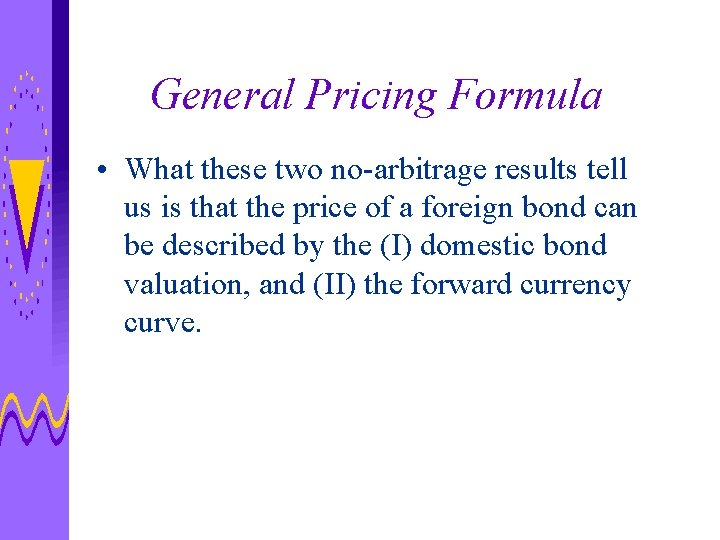General Pricing Formula • What these two no-arbitrage results tell us is that the price of a foreign bond can be described by the (I) domestic bond valuation, and (II) the forward currency curve.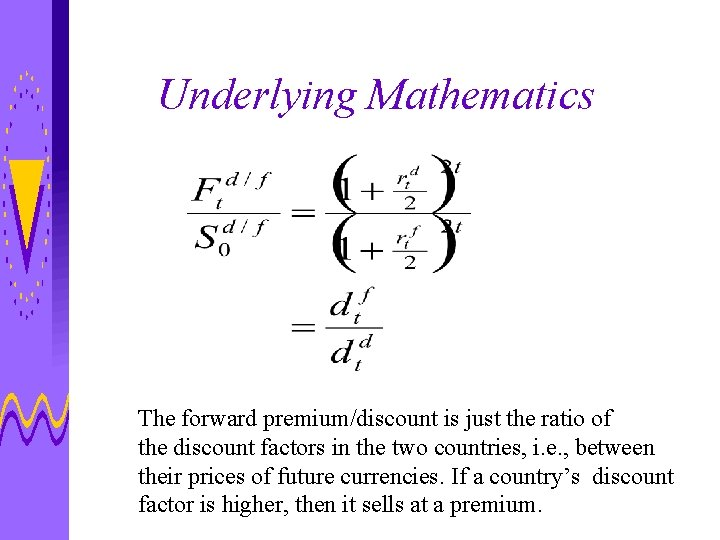Underlying Mathematics The forward premium/discount is just the ratio of the discount factors in the two countries, i. e. , between their prices of future currencies. If a country’s discount factor is higher, then it sells at a premium.General Pricing Formula Using this result, then the value of a foreign bond in dollar terms is: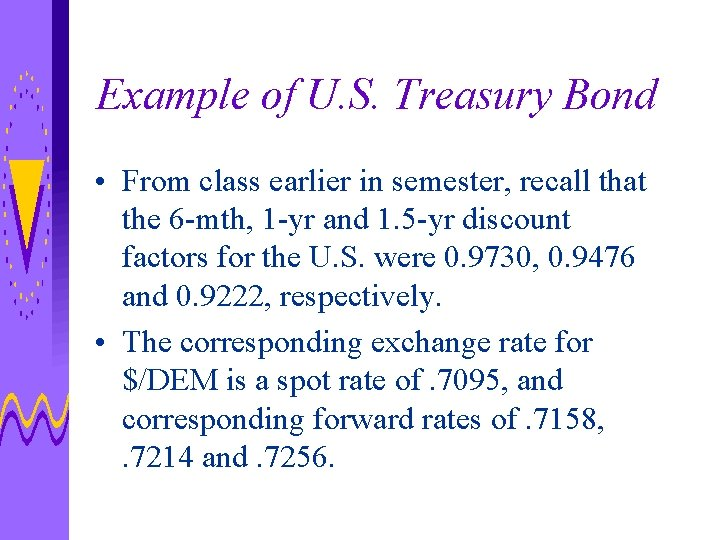Example of U. S. Treasury Bond • From class earlier in semester, recall that the 6 -mth, 1 -yr and 1. 5 -yr discount factors for the U. S. were 0. 9730, 0. 9476 and 0. 9222, respectively. • The corresponding exchange rate for \$/DEM is a spot rate of. 7095, and corresponding forward rates of. 7158, . 7214 and. 7256.Valuing A 1. 5 -Year, 8. 5% T-NoteValuing A 1. 5 -Year, 8. 5% Bund This gives a total value in DEM of 10, 658. 78. Why?Intuition • What happened if the prices of these bonds were different? – Translate the German bund into a U. S. bond by converting future DEM cash flows into US \$. – Take US\$ and discount them at U. S. rates. If this value is different then the bund value times the \$/DEM exchange rate, you have arbitrage!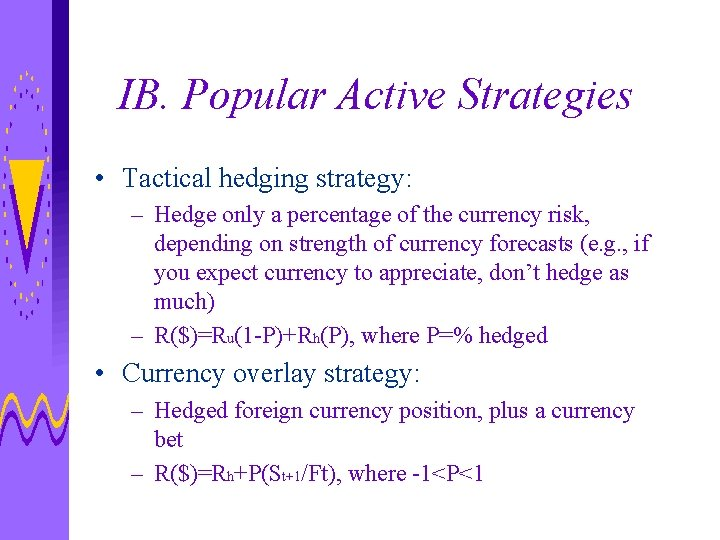IB. Popular Active Strategies • Tactical hedging strategy: – Hedge only a percentage of the currency risk, depending on strength of currency forecasts (e. g. , if you expect currency to appreciate, don’t hedge as much) – R(\$)=Ru(1 -P)+Rh(P), where P=% hedged • Currency overlay strategy: – Hedged foreign currency position, plus a currency bet – R(\$)=Rh+P(St+1/Ft), where -1<P<1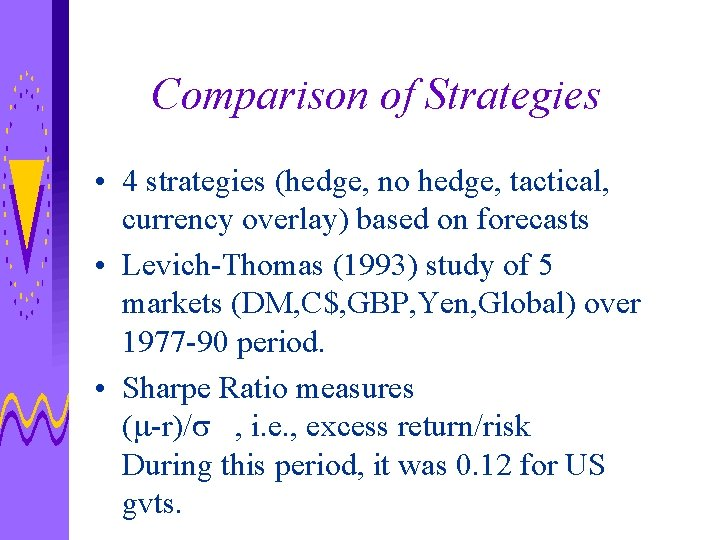Comparison of Strategies • 4 strategies (hedge, no hedge, tactical, currency overlay) based on forecasts • Levich-Thomas (1993) study of 5 markets (DM, C\$, GBP, Yen, Global) over 1977 -90 period. • Sharpe Ratio measures (m-r)/s , i. e. , excess return/risk During this period, it was 0. 12 for US gvts.Sharpe Ratios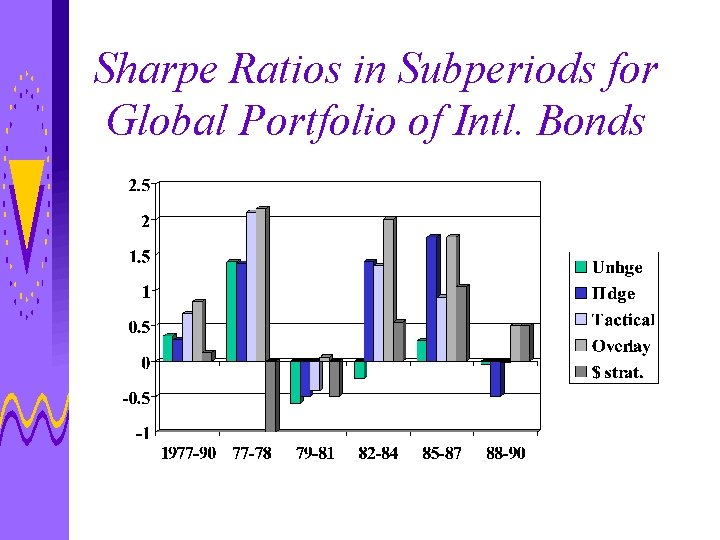Sharpe Ratios in Subperiods for Global Portfolio of Intl. Bonds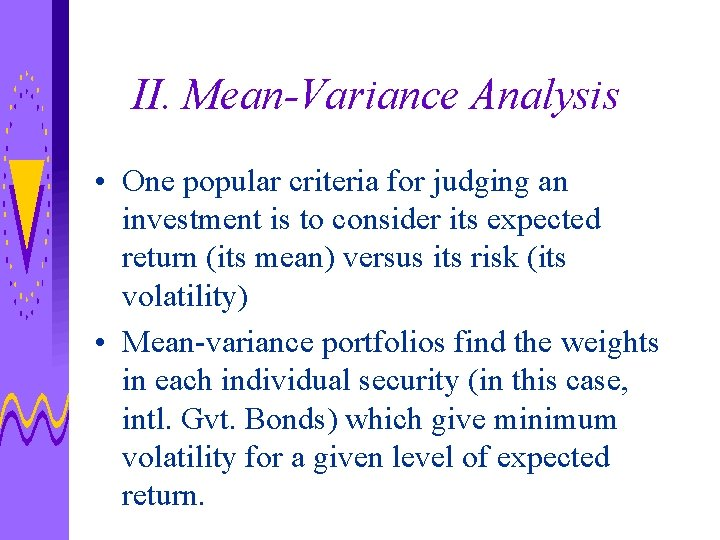II. Mean-Variance Analysis • One popular criteria for judging an investment is to consider its expected return (its mean) versus its risk (its volatility) • Mean-variance portfolios find the weights in each individual security (in this case, intl. Gvt. Bonds) which give minimum volatility for a given level of expected return.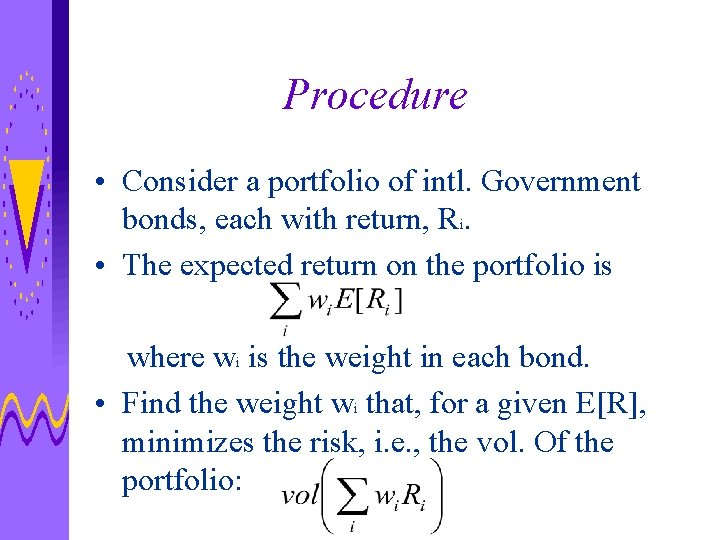Procedure • Consider a portfolio of intl. Government bonds, each with return, Ri. • The expected return on the portfolio is where wi is the weight in each bond. • Find the weight wi that, for a given E[R], minimizes the risk, i. e. , the vol. Of the portfolio: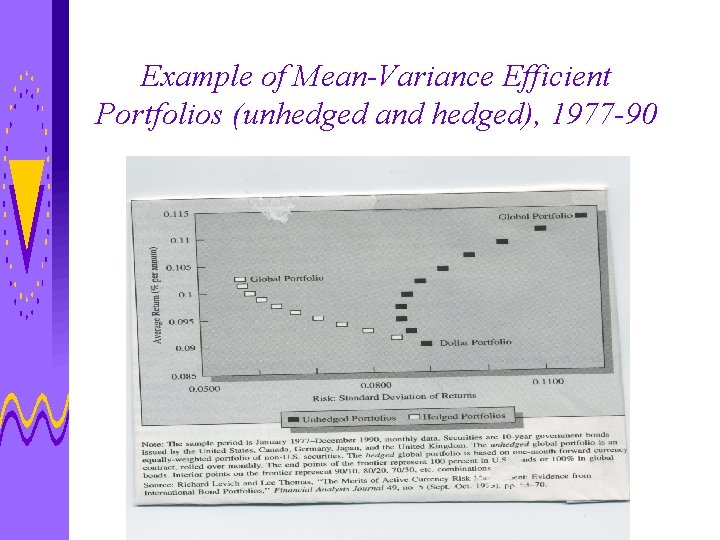Example of Mean-Variance Efficient Portfolios (unhedged and hedged), 1977 -90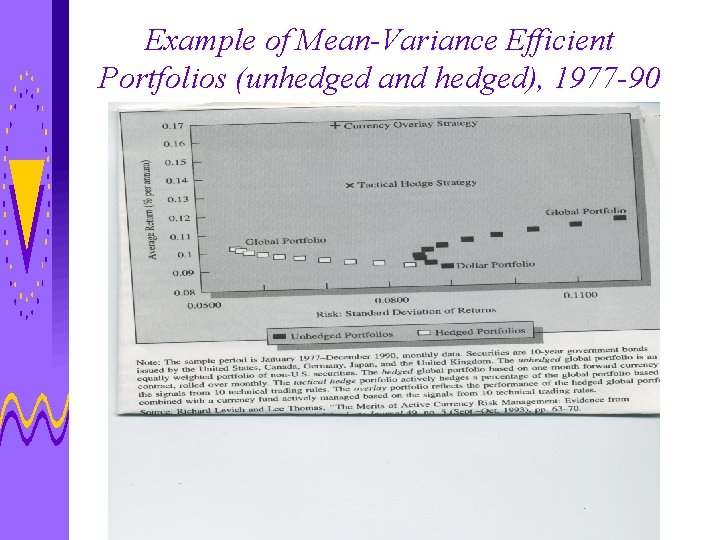Example of Mean-Variance Efficient Portfolios (unhedged and hedged), 1977 -90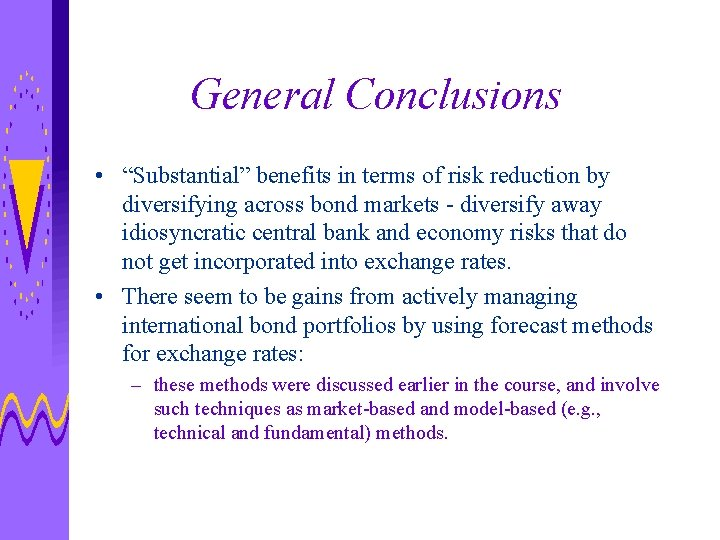General Conclusions • “Substantial” benefits in terms of risk reduction by diversifying across bond markets - diversify away idiosyncratic central bank and economy risks that do not get incorporated into exchange rates. • There seem to be gains from actively managing international bond portfolios by using forecast methods for exchange rates: – these methods were discussed earlier in the course, and involve such techniques as market-based and model-based (e. g. , technical and fundamental) methods.